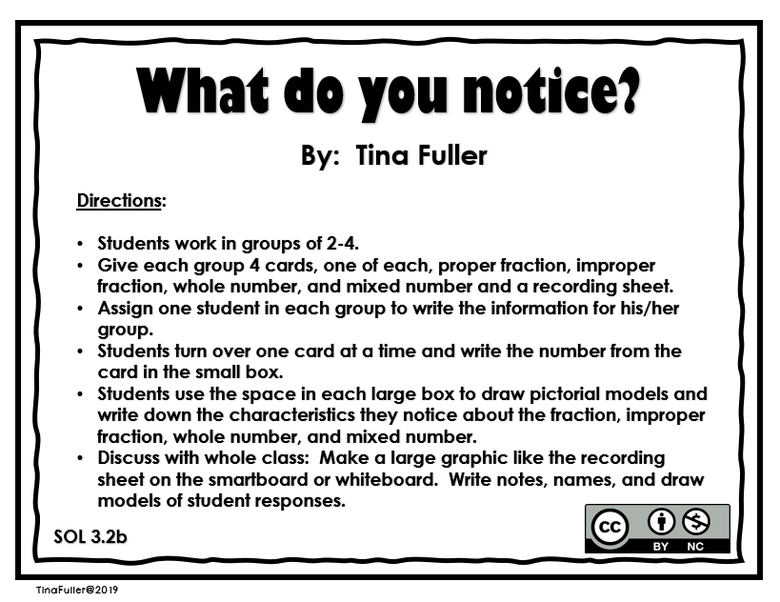Author:
Tina Fuller
Subject:
Mathematics
Material Type:
Activity/Lab
Level:
Upper Primary
3
Tags:
Collaboration, Discussion, Fraction, Math, Small Group
Language:
English
Media Formats:

# What do you notice?## Overview

I created this activity to review proper fractions and whole numbers.  Also, it is a beginning lesson for improper fractions and mixed numbers.  This activity supports sol 3.2b, represent fractions and mixed numbers with models and symbols.  This activity begins in small groups of 2-4 students.  The teacher gives 4 cards to each group, one each of proper fraction, whole number, improper fraction, and mixed number and a recording sheet.  The teacher directs the students to work together to write characteristics about the numbers on the cards and draw any models they can but does not tell them the names of improper fractions and mixed numbers.  Activities like this one gives students opportunity for productive struggle by allowing them to collaborate without too much help from the teacher, it is more rigorous, and the result is students have a deeper understanding.

What do you notice?

I created this activity to review proper fractions and whole numbers.  Also, it is a beginning lesson for improper fractions and mixed numbers.  This activity supports sol 3.2b, represent fractions and mixed numbers with models and symbols.  Prior to this lesson, we studied proper fractions and determined the numerator and denominator.  We used manipulatives (fraction circles for models and skittles/mini erasers for sets).  Also, we drew models and sets to represent proper fractions.  This activity begins in small groups of 2-4 students.  The teacher gives 4 cards to each group, one each of proper fraction, whole number, improper fraction, and mixed number and a recording sheet.  The teacher tells the students to work together to write characteristics about the numbers on the cards and draw any models they can but does not tell them the names of improper fractions and mixed numbers.  Activities like this one gives students opportunity for productive struggle by allowing them to collaborate without too much help from the teacher, it is more rigorous, and the result is students have a deeper understanding.

Materials:

-Cards of proper fraction, whole number, improper fraction, and mixed number (copy on cardstock    and laminate for future use).

-Recording sheet (one for each group)

Directions:

• Students work in groups of 2-4.
• Give each group 4 cards, one of each, proper fraction, improper fraction, whole number, and mixed number and a recording sheet.
• Assign one student in each group to write the information for his/her group.
• Students turn over one card at a time and write the number from the card in the small box.
• Students use the space in each large box to draw pictorial models and write down the characteristics they notice about the proper fraction, improper fraction, whole number, and mixed number.
• Discuss with whole class:  Make a large graphic like the recording sheet on the smartboard or whiteboard.  Write notes, names, and draw models of student responses.